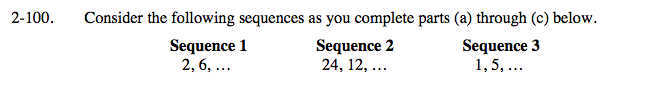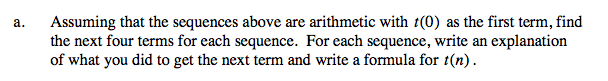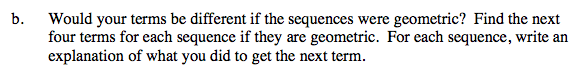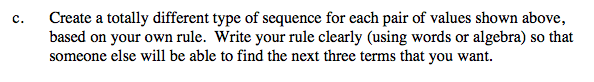### Home > A2C > Chapter 2 > Lesson 2.1.7 > Problem2-100

2-100.Sequence 1:

6 − 2 = 4

Add 4 to each term to get the next.

The next 4 terms are 10, 14, 18, 22.

t(n) = 4n + 2Sequence 1:

$\frac{6}{2}=3$

Multiply each term by 3 to get the next.

The next 4 terms are 18, 54, 162, 486.